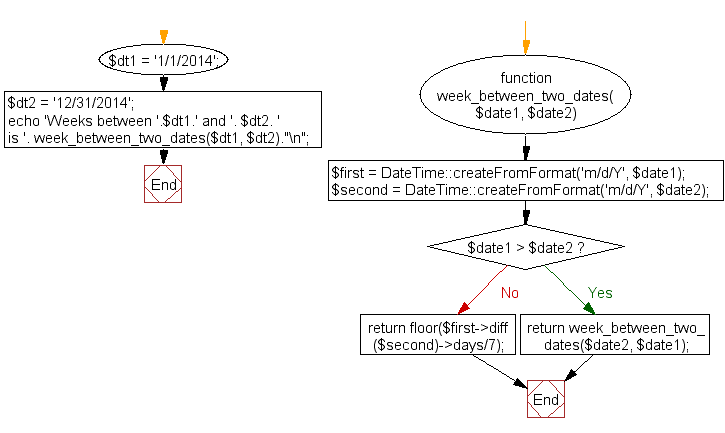﻿ PHP Date Exercise: Calculate weeks between two dates - w3resource# PHP Date Exercises : Calculate weeks between two dates

## PHP date: Exercise-19 with Solution

Write a PHP script to calculate weeks between two dates.

Sample Solution:

PHP Code:

``````<?php
function week_between_two_dates(\$date1, \$date2)
{
\$first = DateTime::createFromFormat('m/d/Y', \$date1);
\$second = DateTime::createFromFormat('m/d/Y', \$date2);
if(\$date1 > \$date2) return week_between_two_dates(\$date2, \$date1);
return floor(\$first->diff(\$second)->days/7);
}

\$dt1 = '1/1/2014';
\$dt2 = '12/31/2014';
echo 'Weeks between '.\$dt1.' and '. \$dt2. ' is '. week_between_two_dates(\$dt1, \$dt2)."\n";
?>
```
```

Sample Output:

```Weeks between 1/1/2014 and 12/31/2014 is 52
```

Flowchart :PHP Code Editor:

Have another way to solve this solution? Contribute your code (and comments) through Disqus.

What is the difficulty level of this exercise?

﻿

## PHP: Tips of the Day

SQL injection that gets around mysql_real_escape_string()

Consider the following query:

```\$iId = mysql_real_escape_string("1 OR 1=1");
\$sSql = "SELECT * FROM table WHERE id = \$iId";
```

mysql_real_escape_string() will not protect you against this. The fact that you use single quotes (' ') around your variables inside your query is what protects you against this. The following is also an option:

```\$iId = (int)"1 OR 1=1";
\$sSql = "SELECT * FROM table WHERE id = \$iId";
```

Ref : https://bit.ly/32q3bJ7